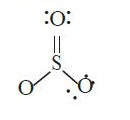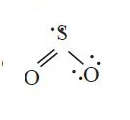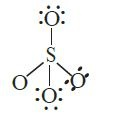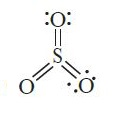# NEET Exams : Previous Year Chemistry Questions Chemical Bonding

5. Which of the following, set of molecules will have zero dipole moment? (NEET 2020)

(a) Ammonia, beryllium difluoride, water, 1, 4-dichlorobenzene

(b) Boron trifluoride, hydrogen fluoride, carbon dioxide, 1, 3-dichlorobenzene

(c) Nitrogen trifluoride, beryllium difluoride, water, 1, 3-dichlorobenzene

(d) Boron trifluoride, beryllium difluoride, carbon dioxide, 1, 4-dichlorobenzen

6. Which of the following is the correct order of dipole moment?  (NEET 2019)

(a) NH3 < BF3 < NF3 < H2O

(b) BF3 < NF3 < NH3 < H2O

(c) BF3 < NH3 < NF3 < H2O

(d) H2O < NF3 < NH3 < BF3

7. The species, having bond angles of 120° is                             (NEET 2017)

(a) ClF3                     (b) NCl3

(c) BCl3                     (d) PH3

8. Consider the molecules CH4, NH3 and H2O. Which of the given statements is false?                                                                                           (NEET-I 2016)

(a) The H — O — H bond angle in H2O is small than the H — N — H bond angle in NH3.

(b) The H — C — H bond angle in CH4 is larger than the H — N — H bond angle in NH3.

(c) The H — C — H bond angle in CH4, the H — N — H bond angle in NH3, and the H — O — H bond angle in H2O are all greater than 90°.

(d) The H — O — H bond angle in H2O is larger than the H — C — H bond angle in CH4.

9. Which of the following molecules has the maximum dipole moment?  (2014)

(a) CO2                    (b) CH4

(c) NH3                   (d) NF3

10. The correct order of increasing bond length of C – H, C – O, C – C and C C  is (2011)

(a) C – H < C = C < C – O < C – C

(b) C – C < C = C < C – O < C – H

(c) C – O < C – H < C – C < C = C

(d) C – H < C – O < C – C < C = C

11. Which of the following structures is the most preferred and hence of lowest
energy for SO3?                                                      (2011)

(a)(b)(c)(d)12. The correct order of increasing bond angles in the  following triatomic species is                                                                                      (2008)

(a) NO2+ < NO2 < NO2             (b) NO2+ < NO2 < NO2

(c) NO2 < NO2+ < NO2             (d) NO2 < NO2 < NO2+

14.
The electronegativity difference between N and F is greater than that between N
and H yet the dipole moment of NH3 (1.5 D) is larger than that of NF3
(0.2 D). This is because          (2006)

(a)
in NH3 the atomic dipole and bond dipole are in the opposite
directions whereas in NF3 these are in the same direction

(b)
in NH3 as well as in NF3 the atomic dipole andbond dipole
are in the same direction

(c)
in NH3 the atomic dipole and bond dipole are in the same direction
whereas in NF3 these are in opposite directions

(d)
in NH3 as well as in NF3 the atomic dipole and bond
dipole are in opposite directions.

16. The correct sequence of increasing covalent character is represented by (2005)

(a) LiCl < NaCl < BeCl2

(b) BeCl2 < LiCl < NaCl

(c) NaCl < LiCl < BeCl2

(d) BeCl2 < NaCl < LiCl

17. Which of the following would have a permanent dipole moment? (2005)

(a) SiF4                            (b) SF4

(c) XeF4                           (d) BF3

23. In the structure of ClF3, the number of lone pairs of electrons on central atom ‘Cl is’                                                                      (NEET 2018)

(a) one                                       (b) two

(c) four                                      (d) three

24. Predict the correct order among the following:                      (NEET 2016)

(a) Bond pair – bond pair > lone pair – bond pair > Lone pair – lone pair

(b) Lone pair – bond pair > bond pair – bond pair > Lone pair – lone pair

(c) Lone pair – lone pair > lone pair – bond pair > Bond pair – bond pair

(d) Lone pair – lone pair > bond pair – bond pair > Lone pair – bond pair

25. Which of the following species contains three bond pairs and one lone pair around the central atom?                                                       (2012)

(a) H2O                                      (b) BF3

(c) NH 2                                   (d) PCl3

26. Which of the following is not a correct statement?                 (2006)

(a) Multiple bonds are always shorter than Corresponding single bonds.

(b) The electron-deficient molecules can act as Lewis acids.

(c) The canonical structures have no real existence.

(d) Every AB5 molecule does in fact have square

27. Which of the following is not isostructural with SiCl4?                    (2006)

(a) NH4+                          (b) SCl4

(c) SO42–                          (d) PO43–

28. In which of the following molecules all the bonds are not equal?           (2008)

(a) NF3                (b) ClF3

(c) BF3                (d) AlF3

36. Which of the following species contains equal number of σ and π -bonds?(2015)

(a) (CN)2     (b) (CH)2(CN)2      (c) HCO3    (d) XeO

37. Which one of the following molecules contains no π bond?            (2013)

(a) SO2        (b) NO2

(c) CO2       (d) H2O

44. Which of the following pairs of compounds is isoelectronic and isostructural? (2017)

(a) TeI2, XeF2                            (b) IBr2, XeF2

(c) IF3 , XeF2                             (d) BeCl2, XeF2

45. The hybridizations of atomic orbitals of nitrogen in NO+, NOand NH+ respectively                                                                                  (2016)

(a) sp, sp3 and sp2         (b) sp2, sp3 and sp

(c) sp, sp2 and sp3            (d) sp2, sp and sp3

46. Which of the following pairs of ions is isoelectronic and isostructural? (NEET-II 2016)

(a) CO3 2–, NO3                                 (b) ClO3 , CO3 2-

(c) SO32–, NO3                                   (d) ClO3, SO32–

47. The correct geometry and hybridization for XeF are             (NEET-II 2016)

(a) octahedral, sp3d2

(b) trigonal bipyramidal, sp3d

(c) planar triangle, sp3d3

(d) square planar, sp3d2.

48. Among the following, which one is a wrong statement?        (NEET-II 2016)

(a) PH5 and BiCl5 do not exist.

(b) pπ-dπ bonds are present in SO2.

(c) SeF4 and CH4 have same shape.

(d) I3+ has bent geometry.

49. In which of the following pairs, both the species are not isostructural? (2015)

(a) Diamond, Silicon carbide

(b) NH3, PH3

(c) XeF4, XeO4

(d) SiCl4, PCl+4

50. Maximum bond angle at nitrogen is present in which of the following? (2015)

(a) NO2+                                    (b) NO3

(c) NO2                                      (d) NO2